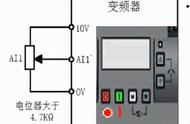# 西门子MM4202.2KW变频器用端子控制启停外部模拟量（0-10v）信号控制频率怎么接线和设置参数P0100=0

P0205=0

P0300=1

P0304=400

P0305=4.7  电机铭牌上的额定电流

P0307=2.2   电机的额定功率

P0310=50

P0311=1425   铭牌上的电机额定转速

P0700=2     外部启停

P1000=2     外部启停

P1080=0

P1082=50

P1120=10     加速时间

P1121=10     减速时间

P1135=5

P1300=20

P3900=1

DIP2小开关是设置频率的开关，选择50hz还是60hz标准。